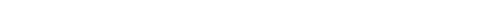## Question of the Week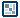Easing - Page 1
by Voetsjoeba  |  9 December 2006

Easing is a way of moving from one location to another, so that the speed of the object that moves along its track varies. This tutorial will deal with a specific type of easing called ‘Easing Out’; easing in a way so that the object’s speed decreases as it nears its destination. The complement of Easing Out is Easing In, and since they are both similar, I will mention Easing In only in passing towards the end of the tutorial.

1. Introduction to Easing
Consider the following two examples to get a good grasp of what the difference is between the two. Both represent an object moving from the left towards a destination on the right, but in two different ways. The first one uses no easing at all ( a constant speed ) to move to its destination, the second uses ‘Easing Out’ to move to its destination. From now on I will refer to ‘Easing Out’ as simply ‘easing’, for the sake of consistency and readability.

The above example does not use any easing. Notice how the square's speed stays constant throughout the movement.

On the other hand, the above square contains a slight easing. You should see the square start to decelerate as it nears its destination.

So, easing as we’ll discuss it here is the way of moving where an object slows down as it nears its goal. The key to implementing this in Flash might be simpler than you thought. What happens is basically you take the distance between the object and its destination, divide it by a number greater than 1, and you now have a distance that is smaller. We then move our object to be at the newly calculated distance from its destination, and repeat this reduction as long as necessary.

Why does this make it ease? Well, since we’re dividing, the greater the distance is between the object and its destination, the greater the difference in calculated distance will be in absolute terms. Put otherwise: the closer the object gets to its goal, the smaller the distance to the goal is. The smaller the distance to the goal, the smaller the distance will be that we calculate. The smaller the distance is that we calculate, the less we move the object moves towards its goal. Thus we have easing movement.

That might sound a bit confusing. Consider the following example: we have an object that is currently at position 0, and we want to ease it to position 120. We must first pick a number to divide each distance by, as this will determine the speed of our easing motion. Let’s pick 2. We can now follow these steps:

1. Take the distance between the object’s current position and its destination

2. Divide this distance by 2

3. Move the object towards its destination, so that it is the distance we calculated in step 2 away from it.

4. Repeat Step 1

Indeed, this is a loop, every iteration through it updating the object’s position. To clarify how it works, here’s a visual representation. The line on the left indicates the object’s starting position (0), and the line on the right indicates the object’s destination (120). The orange line represents the object’s new position, calculated by dividing the distance between current position and destination by 2.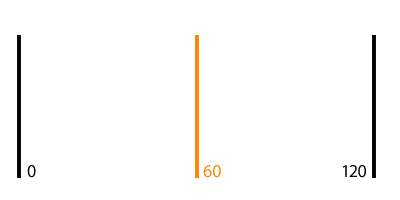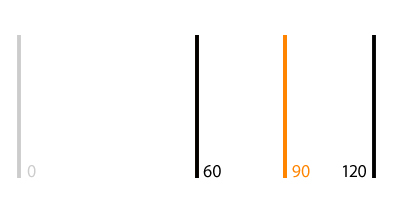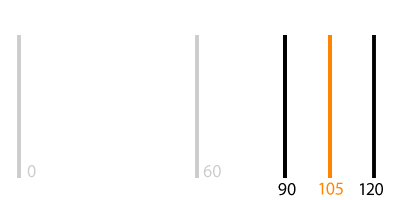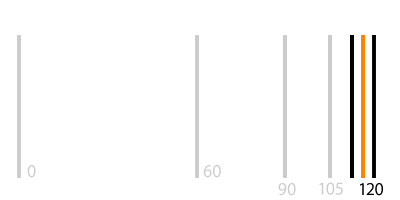Considering that you can go on and on, always dividing the distance by 2 (don’t worry about ending the loop yet), we get the following movement pattern: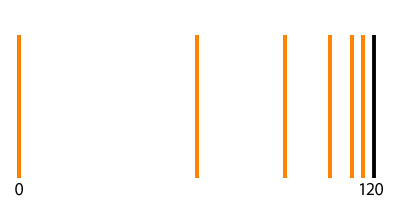Now that you have an understanding of how to think about easing out effects, let's delve into how easing can be implemented in Flash.

Onwards to the next page!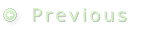1 | 2 | 3 | 4 | 5 | 6